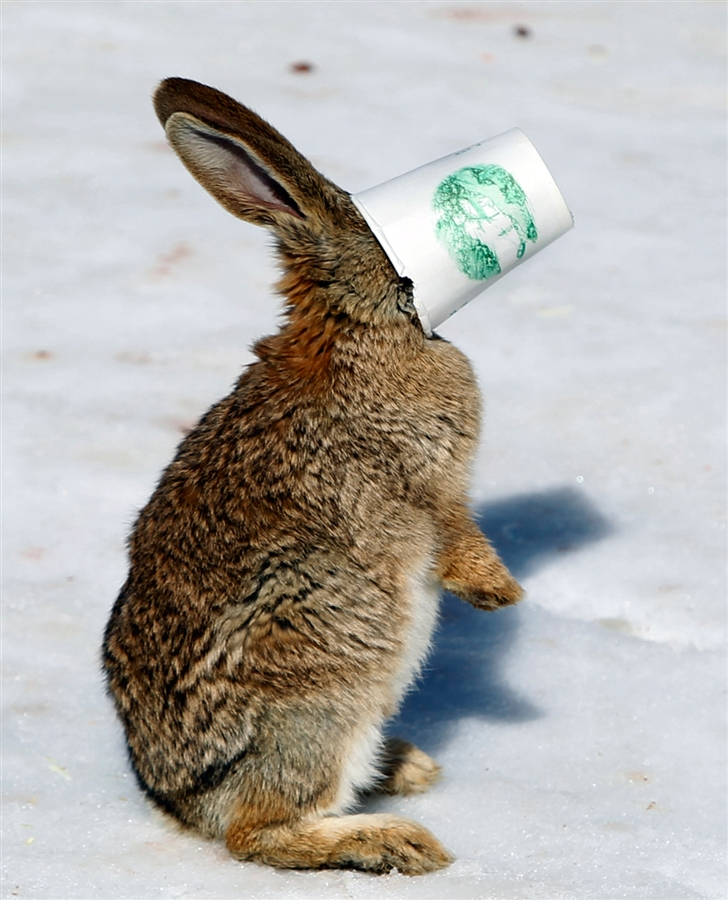## Enumerations, Bitwise Operators, Shiftiness

I like enumerations. They’re really useful for writing clear code and they’re also really easy to use. Like this:

```public enum TvChannels
{
BbcOne = 0,
BbcTwo = 1,
ItvOne = 2,
ChannelFour = 3
}```

Right?

Wrong. Enumerations can be decorated with the [Flags] attribute, which allows them to be combined. This allows them to be grouped together:

`var BbcChannels = TvChannels.BbcOne | TvChannels.BbcTwo`

The variable BbcChannels is defined as a bitwise OR between BbcOne and BbcTwo. The way we previously defined our enum values presents a problem however. The values must be multiples of two, otherwise the bitwise operations will yield incorrect results. The enumeration should instead be defined as follows:

```public enum TvChannels
{
None = 0
BbcOne = 1,
BbcTwo = 2,
ItvOne = 4,
ChannelFour = 8
}```

There are two points to note here.

Firstly, the multiples of two allow the OR operations to work. In binary, the value of BbcOne is 0001, and that of BbcTwo is 0010. The result of an OR operation between the two (defining the variable BbcChannels) is then 0011.

This allows us to then check the BbcChannels for other enumeration values, eg

`var IsBbcChannel = (BbcChannels & TvChannels.BbcOne) == TvChannels.BbcOne`

This will be True, because the bitwise AND between BbcChannels (0011) and BbcOne (0001) is 0001 – ie equal to BbcOne.

The second point is that we have introduced a value of None = 0 at the start of the enumeration. This is because a value of zero cannot be tested for using a bitwise AND in the same way as in the example above; the result would always be zero.

Finally, a bitshift operator can be used to make the enumeration a bit nicer to look at. Because we’re assigning specific bits to each successive element in the enumeration, we can simply bit shift to the left by the appropriate number of times:

```public enum TvChannels
{
None = 0
BbcOne = 1 << 0,
BbcTwo = 1 << 1,
ItvOne = 1 << 2,
ChannelFour = 1 << 3
}```

Lovely.

Here’s a photo of a rabbit with a cup on its head: